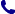• Kontakt
• Newsletter Zapisz się po informacje o nowościach i specjalnych ofertach. Administrator danych: ABE-IPS sp. z o.o.
Wybierz interesujące Cię kategorie
Wyrażam zgodę na przetwarzanie moich danych osobowych w celach marketingowych, tym samym akceptuję Regulamin Newslettera i Politykę prywatności.
Wyrażam zgodę na przesyłanie informacji handlowych drogą elektroniczną.
Wypisz się
• Klienci instytucjonalni
• Dostawa
• O nas
Dostęp on-line

# Książki

0.00 PLN
Schowek (0)
 Schowek jest pusty

## Change and Variations: A History of Differential Equations to 1900

 Autorzy Wydawnictwo Springer, Berlin Data wydania Liczba stron 419 Forma publikacji książka w miękkiej oprawie Język angielski ISBN 9783030705749 Kategorie Historia matematyki
276.15 PLN (z VAT)
\$62.12 / €59.21 / £51.40 /
Zapowiedź
Chcesz otrzymać informację, gdy książka będzie dostępna do kupna? Podaj swój adres e-mail, na który prześlemy powiadomienie:
 E-mail Wyślij
Do schowka

## Opis książki

This book presents a history of differential equations, both ordinary and partial, as well as the calculus of variations, from the origins of the subjects to around 1900. Topics treated include the wave equation in the hands of d'Alembert and Euler; Fourier's solutions to the heat equation and the contribution of Kovalevskaya; the work of Euler, Gauss, Kummer, Riemann, and Poincaré on the hypergeometric equation; Green's functions, the Dirichlet principle, and Schwarz's solution of the Dirichlet problem; minimal surfaces; the telegraphists' equation and Thomson's successful design of the trans-Atlantic cable; Riemann's paper on shock waves; the geometrical interpretation of mechanics; and aspects of the study of the calculus of variations from the problems of the catenary and the brachistochrone to attempts at a rigorous theory by Weierstrass, Kneser, and Hilbert. Three final chapters look at how the theory of partial differential equations stood around 1900, as they were treated by Picard and Hadamard. There are also extensive, new translations of original papers by Cauchy, Riemann, Schwarz, Darboux, and Picard.

The first book to cover the history of differential equations and the calculus of variations in such breadth and detail, it will appeal to anyone with an interest in the field. Beyond secondary school mathematics and physics, a course in mathematical analysis is the only prerequisite to fully appreciate its contents. Based on a course for third-year university students, the book contains numerous historical and mathematical exercises, offers extensive advice to the student on how to write essays, and can easily be used in whole or in part as a course in the history of mathematics. Several appendices help make the book self-contained and suitable for self-study.

Change and Variations: A History of Differential Equations to 1900

## Spis treści

1 The First Ordinary Differential Equations.- 2 Variational Problems and the Calculus.- 3 The Partial Differential Calculus.- 4 Rational Mechanics.- 5 Partial Differential Equations.- 6 Lagrange's General Theory.- 7 The Calculus of Variations.- 8 Monge and Solutions to Partial Differential Equations.- 9 Revision.- 10 The Heat Equation.- 11 Gauss and the Hypergeometric Equation.- 12 Existence Theorem.- 13 Riemann and Complex Function Theory.- 14 Riemann and the Hypergeometric Equation.- 15 Schwarz and the Complex Hypergeometric Equation.- 16 Complex Ordinary Differential Equations: Poincaré.- 17 More General Partial Differential Equations.- 18 Green's Functions and Dirichlet's Principle.- 19 Attempts on Laplace's Equation.- 20 Applied Wave Equations.- 21 Revision.- 22 Riemann's Shock Wave Paper.- 23 The Example of Minimal Surfaces.- 24 Partial Differential Equations and Mechanics.- 25 Geometrical Interpretations of Mechanics.- 26 The Calculus of Variations in the 19th Century.- 27 Poincaré and Mathematical Physics.- 28 Elliptic Equations and Regular Variational Problems.- 29 Hyperbolic Equations.- 30 Revision.- 32 Translations.- A Newton's Principia Mathematica.- B Characteristics.- C First-order Non-linear Equations.- D Green's Theorem and Heat Conduction.- E Complex Analysis.- F Möbius Transformations.- G Lipschitz and Picard.- H The Assessment.- Bibliography.- Index.

## Polecamy również książki801 777 223